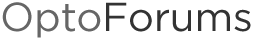# Does OptoScript support indirect addressing of Variables?

For an Example…

For [B]x[/B] = 1 to 4
MyTag[B]x[/B] = 1 // Indirectly addressed variable’s value assignment.
Next

MyTag[B]x[/B] would reference variables MyTag1, MyTag2, MyTag3, etc.

MyTag1 =1
MyTag2 =1
MyTag3 =1
MyTag4 =1

Values for “MyTagx” will almost always will never be set in a loop at the same time, but just wanted to illustrate the idea to see if this sort of thing is possible with Optoscript.

Thanks

Indirectly, using GetPointerFromName as I mention in [B][U]this post on Eval()[/U] [/B]and [URL=“http://www.opto22.com/community/showthread.php?t=196&p=568&viewfull=1#post568”][U][B]this post too[/B][/U]; but those depend on the name of your variables NEVER CHANGING which is impossible to guarantee.

Better to use a table and loop on the index. Have you considered that? Or perhaps you want to loop through a set of tables? What are you up to here?

Have you considered defining MyTag as a numeric table?

For x = 1 to 4 Step 1
MyTag[x] = 1;
next

Edit:

For bonus points, you could put a list of more descriptively named variables into a pointer table, and then cycle through the pointers in a similar command:

ptPointer = &aoModule1_1;
ptPointer = &aoModule1_2;
ptPointer = &aoModule2_1;
ptPointer = &aoModule2_2;

For x = 0 to 3 step 1
pPointer = ptPointer[x];
*pPointer = 0; //sets the analog output to zero
next

I guess we are talking about two completely different addressing methods. I started off by providing a bad example (indexed instead of indirect which is what I was wanting). What you are referring to is Indexed addressing. i.e Table/Array access or referencing.

What I am wanting is Indirect Addressing of a variable. For an example in a table say that I have …(does not have to be a table. Can be any valid Variable or I/O Point declaration).

Tag = 0
Tag = 1
Tag = 2
Tag = 500

Say I have another variable (could be a variable or a variable table, Input or output data from I/O racks)

nVariableX = 3

So if i were to indirectly address a variable to get at it is value, Expression would be written as;
[B]
NewVariable = Tag[nVariableX][/B] would give me a value of 500 for the [B]NewVariable[/B]. Which is what the value of Tag is.

So Tag[nVariableX] is [B]INDIRECTLY [/B]referencing Tag based on the [B]value[/B] of nVariableX.

Thanks for the links and Pointers. (No Pun Intended)

Best regards.

The sequence you outlined:

Tag = 2;
Tag = 500;
nVariableX = 3;
NewVariable = Tag[nVariableX]; // NewVariable = 500

is standard functionality. If you want NewVariable to follow the value of Tag if it changes, then NewVariable needs to be a pointer.

pNewVariable = &Tag[nVariableX]; //pNewVariable will always return the current value of Tag.

you could take it further, but it requires two lines of code…

nVariableX = 2; //nothing happens
pNewVariable = &Tag[nVariableX]; //pNewVariable now returns the current value of Tag

I might still not be understanding correctly what you want, so here’s another example with pointers (might be more useful to just post a picture of a rabbit with a pancake on its head):

Let’s say you have i32’s “nX” and “nY”, and pointer “pA”.

nY = 6;
nX = nY; //nX now equals 6
pA = &nX; //pA now returns 6, referencing nX
nX = 5; //pA now returns 5
*pA = nY; //nX now equals 6

Edit:
In Page 18 of this document, it looks like this pointer example matches up to the indirect addressing example, so maybe this isn’t too far off…